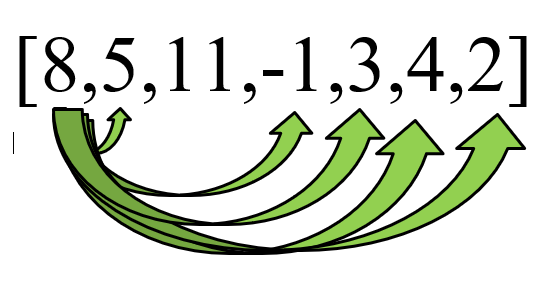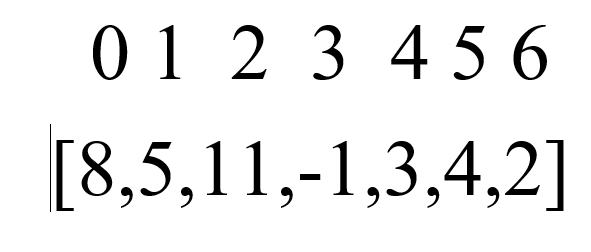# Coding Challenge: Right Smaller Than

Coding Challenges are the bread and butter of getting a software development position as all major companies test you on coding challenges for software development positions. An entire industry is designed around preparing candidates for coding challenges. Coding challenges are difficult and learning how to approach each coding challenge is difficult. I initially didn't like learning coding challenges. However, after a while i began to enjoy learning them as i felt it opened my eyes to common data structures and patterns. This blog will focus on a coding challenge called “Right Smaller Than” which deals with one of the most common data structures called an array. This coding challenge is considered a difficult coding challenge by many. I hope this blog will help other developers learn how to approach to this problem in a step by step fashion.

# The Problem Statement

For every number in an array look to the right of the array and count how many times a number that is smaller than it appears in the array.

Example: Original Array [8,5,11,-1,3,4,2]

returns this array [5,4,4,0,1,1,0]

In this example the first number in the original array is 8 and if we counted the numbers to the right that are smaller than it we will get a total of 5 numbers. If we continue on to the next number in the original array which is 5 we see that there are 4 numbers to the right of 5 that are smaller than it. If we continued this comparison for all numbers than we will eventually get the full array of [5,4,4,0,1,1,0].The number 8 has 5 numbers smaller than it to the right of the array

# Approach to Solving this problem

Solving coding problems like this can be daunting, as human we can easily solve this problem off the top of our head by looking to the right of the number and count how many numbers are smaller than it. Creating a computer function that solves this problem is a lot more difficult as we must understand how computers think in terms of arrays, comparison, counting, storing data and returning the results we are looking for. My approach to solving this problem is first think in terms of how as a human being i would solve this problem and then using my knowledge of programming how i can code this solution so that a computer program can execute the code properly in order to return the correct results. In terms of how we think as human beings compared to how computer programs function is the main difficulty in solving many coding challenges.

# How a Human Being will Solve this Problem

Anyone who understand the problem statement will solve this problem easily. If you were to describe how you solved this problem in regular human terms, you can describe it in the following manner.

“I will look at the first number in the original array and compare all the number to the right of it and see if it is smaller than the first number. I will then create a new array and add the total amount of numbers smaller than it to the new array. I will continue this process with every number in the original array until i reach the last number in the original array. We will finally return the counts for each number in the new array.”

# Solving Problem as a Computer Program

Lets translate this solution from human terms to computer program terms so we can write code that solves this coding challenge. In the previous example i highlighted key terms in order to show how human terms can be translated to computer code. Lets go over each highlighted part.

look at the first number: this means we must access the array through its index. In software development we access array values through their index. Remember that in many program languages we find the place value of an item in array using an index and the first index in an array for many programming languages starts with 0 instead of starting with the number 1. In the original array we will say that the first number 8 is at index of 0.The first value of any array in JavaScript starts with an index of 0.

compare all the number to the right of it and see if it is smaller than the first number: this translates to a comparison operator such as >(greater than) or <(less than) in coding.

create a new array: this means we must create a new array in the computer’s memory where we can store values.

add the total amount of numbers smaller than it to the new array: this indicates we need to utilize a counter (++) that keeps track of the numbers smaller than it and places it inside (.push) the new array.

continue this process with every number in the original array: this indicates we will have to utilize a loop which goes through each number in the original array.

return the counts for each number in the new array: this indicates we must have a return statement in our code in order for us to have the new array with the proper counts.

# Code Solution

Let’s take a look at one possible solution in JavaScript that utilizes both the human terms and computer program terms discussed earlier.

`let array = [8, 5, 11, -1, 3, 4, 2]function rightSmallerThan(array) {const rightSmallerCounts = []for (let i = 0; i < array.length; i++) {let rightSmallerCount = 0for (let j = i + 1; j < array.length; j++) {if (array[j] < array[i])rightSmallerCount++}rightSmallerCounts.push(rightSmallerCount)}return rightSmallerCounts}rightSmallerThan(array)`

# Code Break Down

I like to write out code on a whiteboard in order to practice whiteboarding which many software developers had to do pre-corona virus days for in person interviews. In the images below i break down step by step what each line of code is doing and how in incorporates many of the human/computer terms we discussed.